# MSBSHSE Solutions For Class 8 Maths Part 1 Chapter 8- Quadrilateral: Constructions and Types

## Download the PDF of Maharashtra Board Solutions For Class 8 Maths Part 1 Chapter 8- Quadrilateral: Constructions and Types.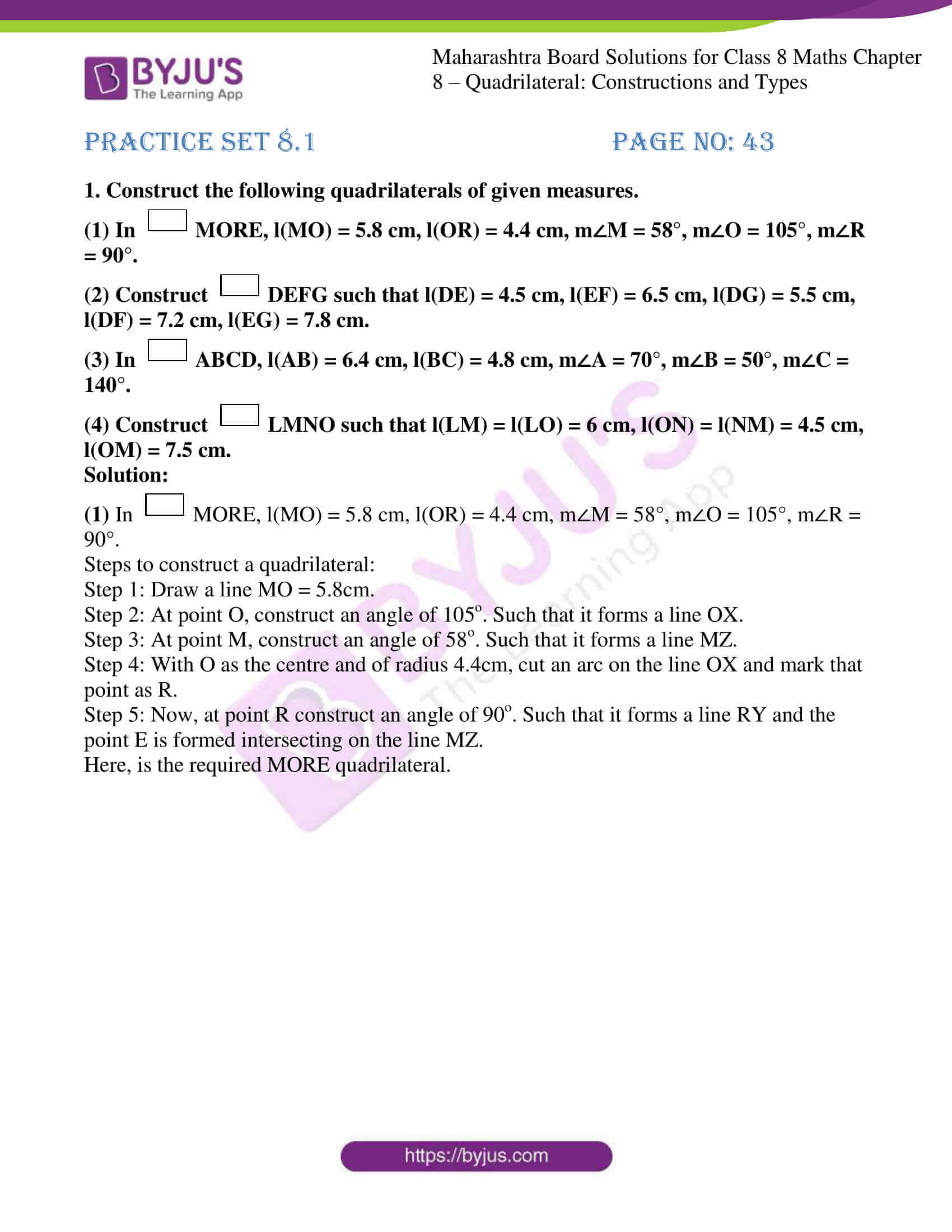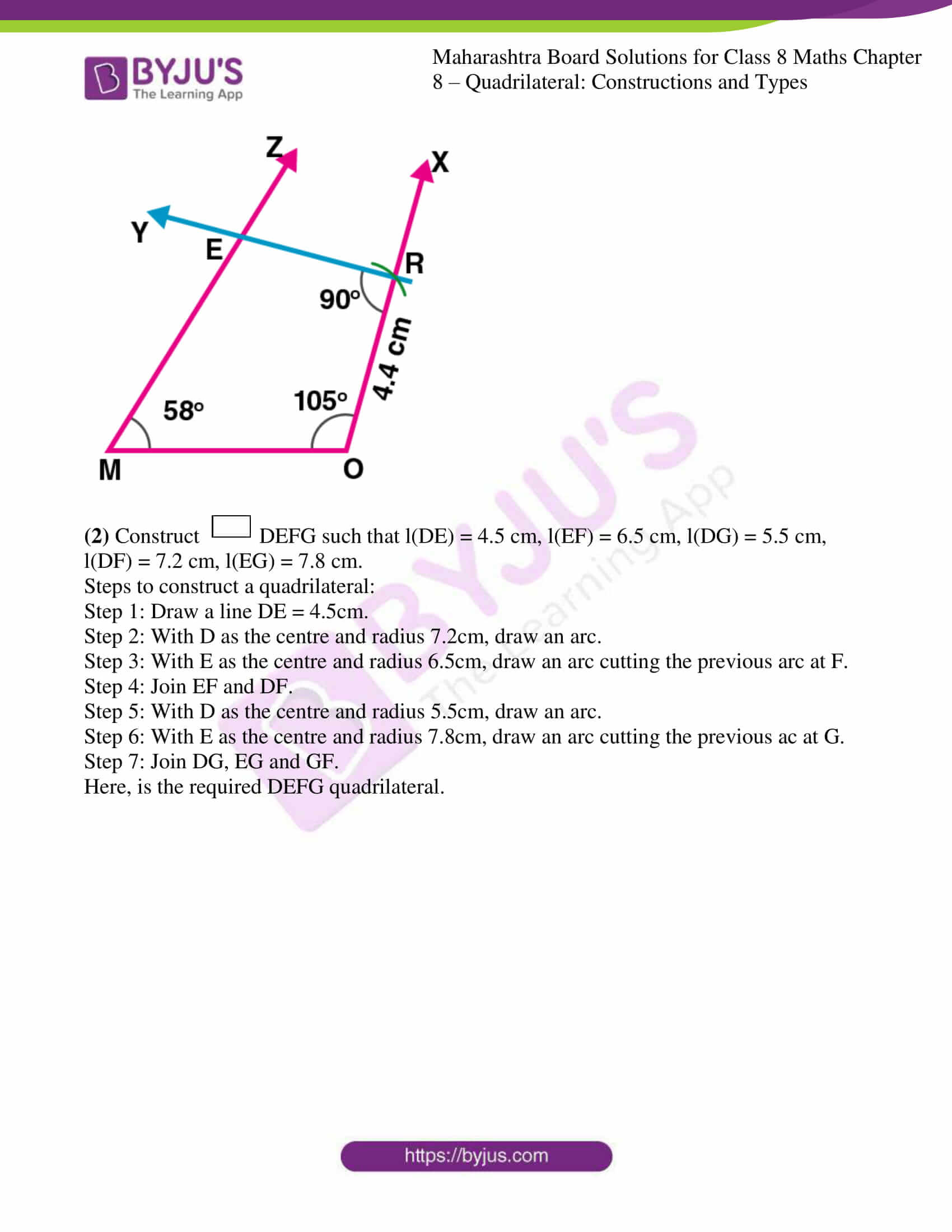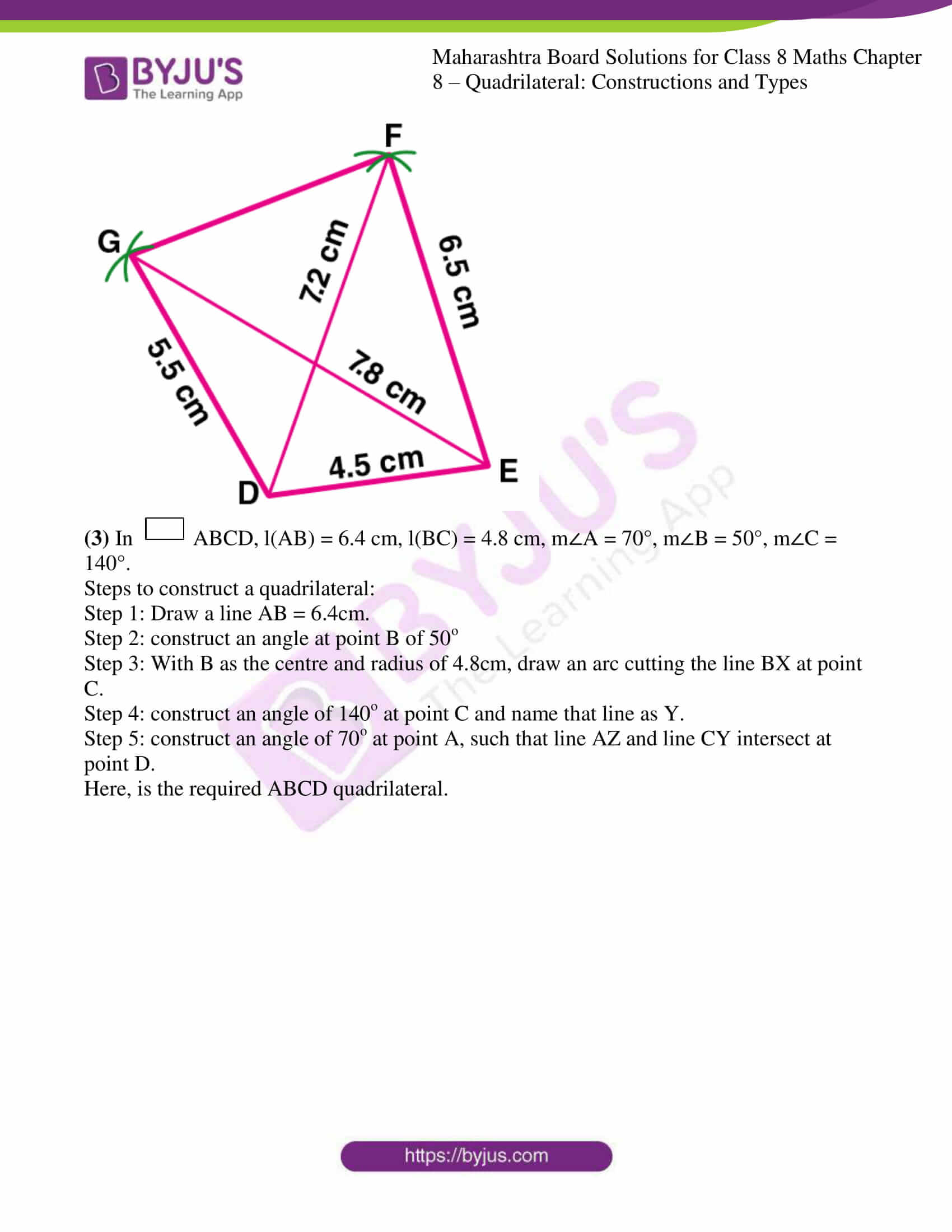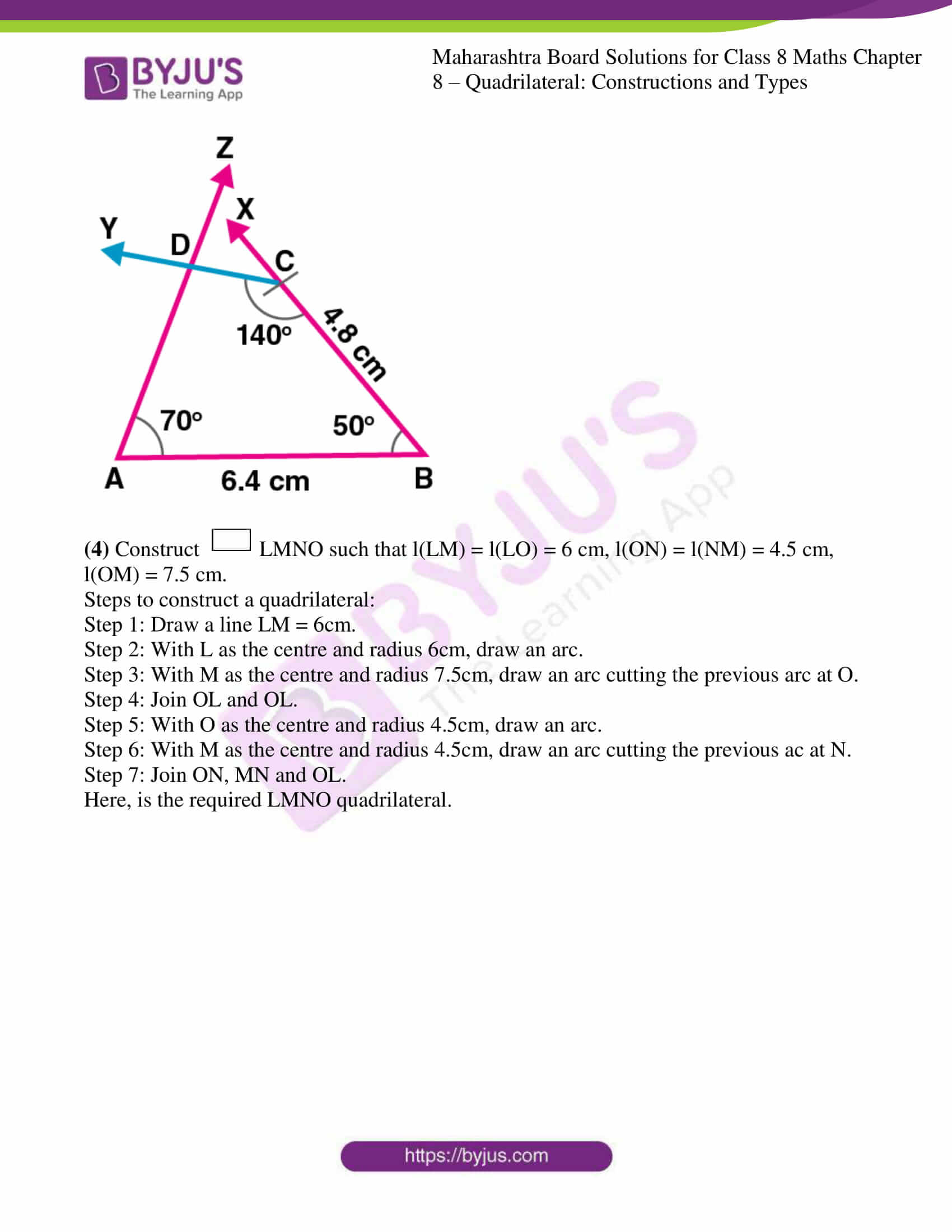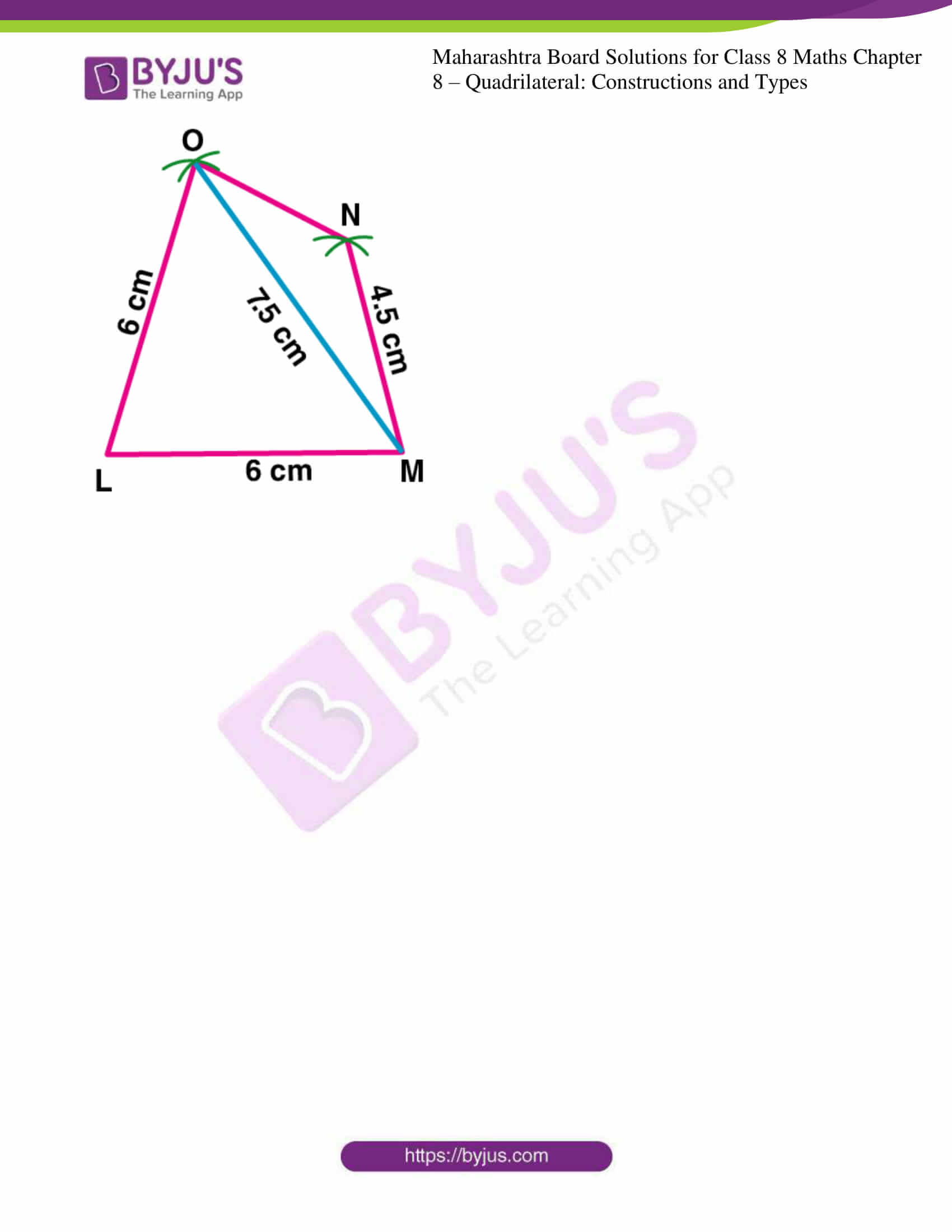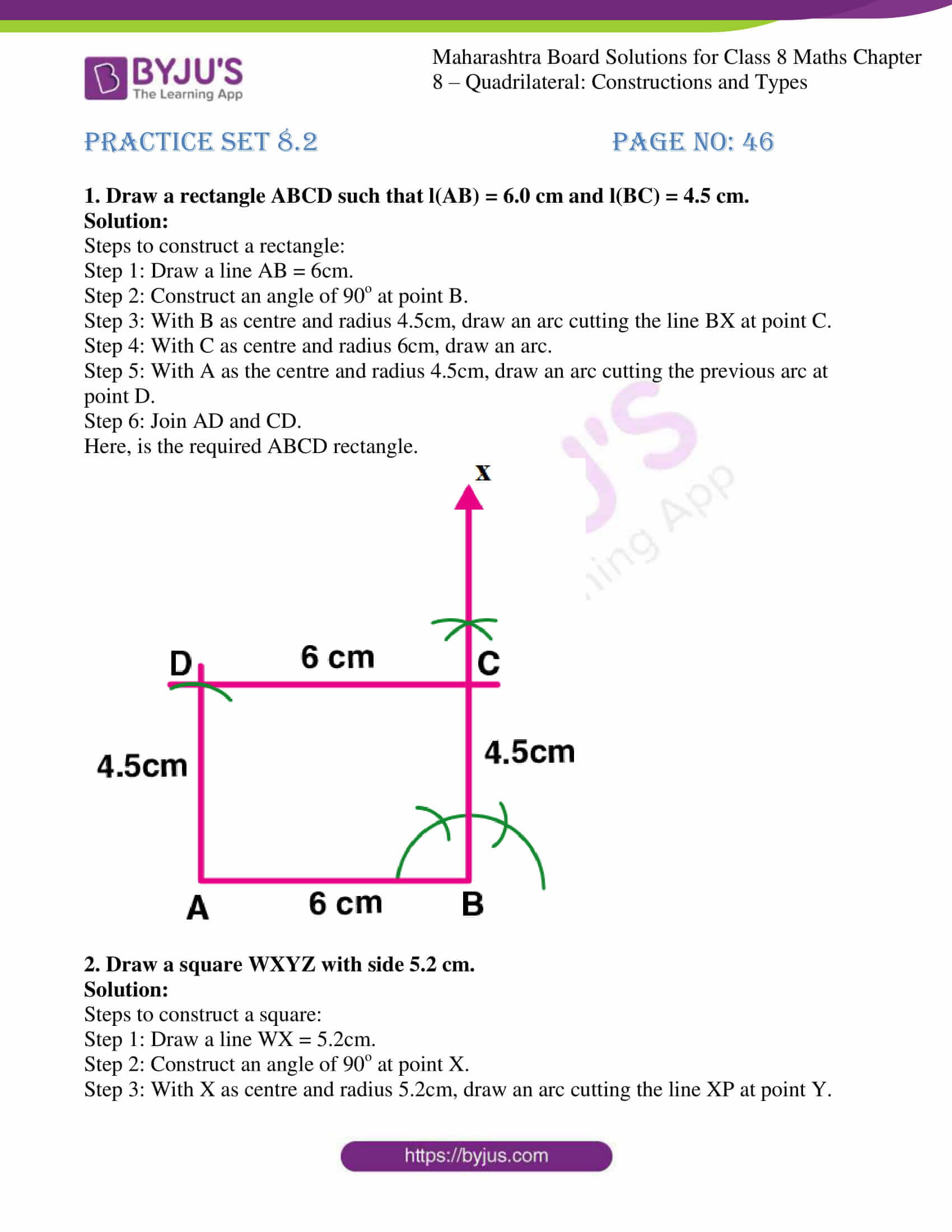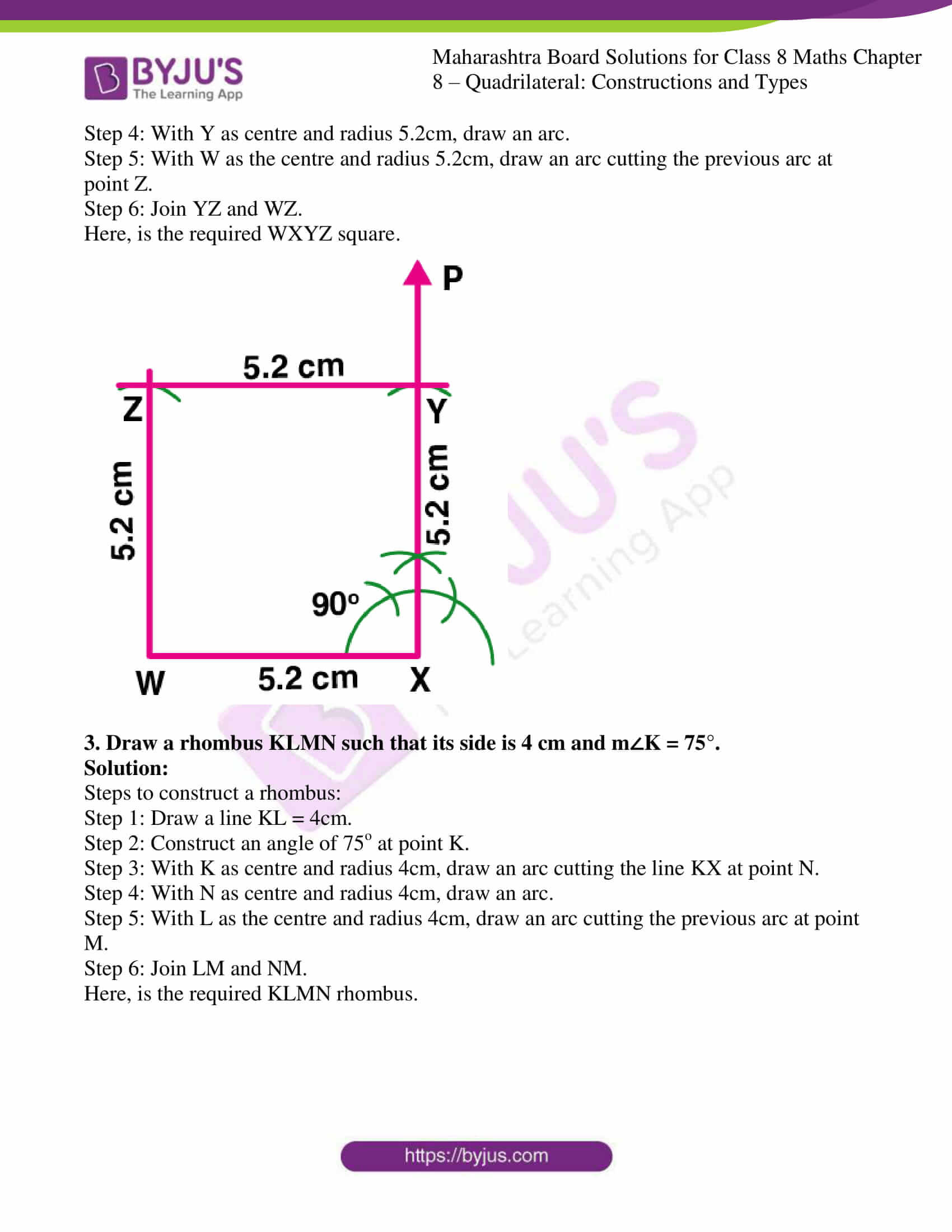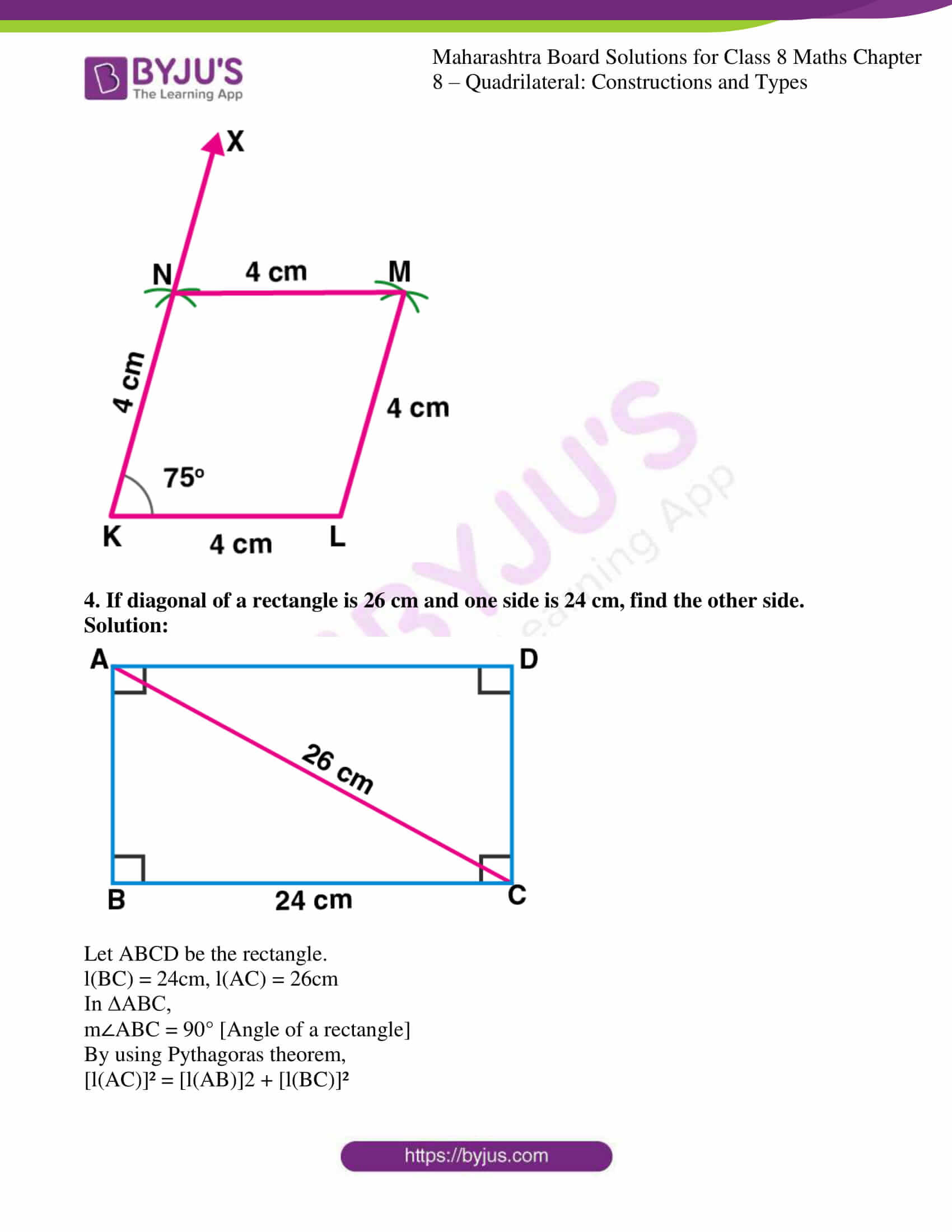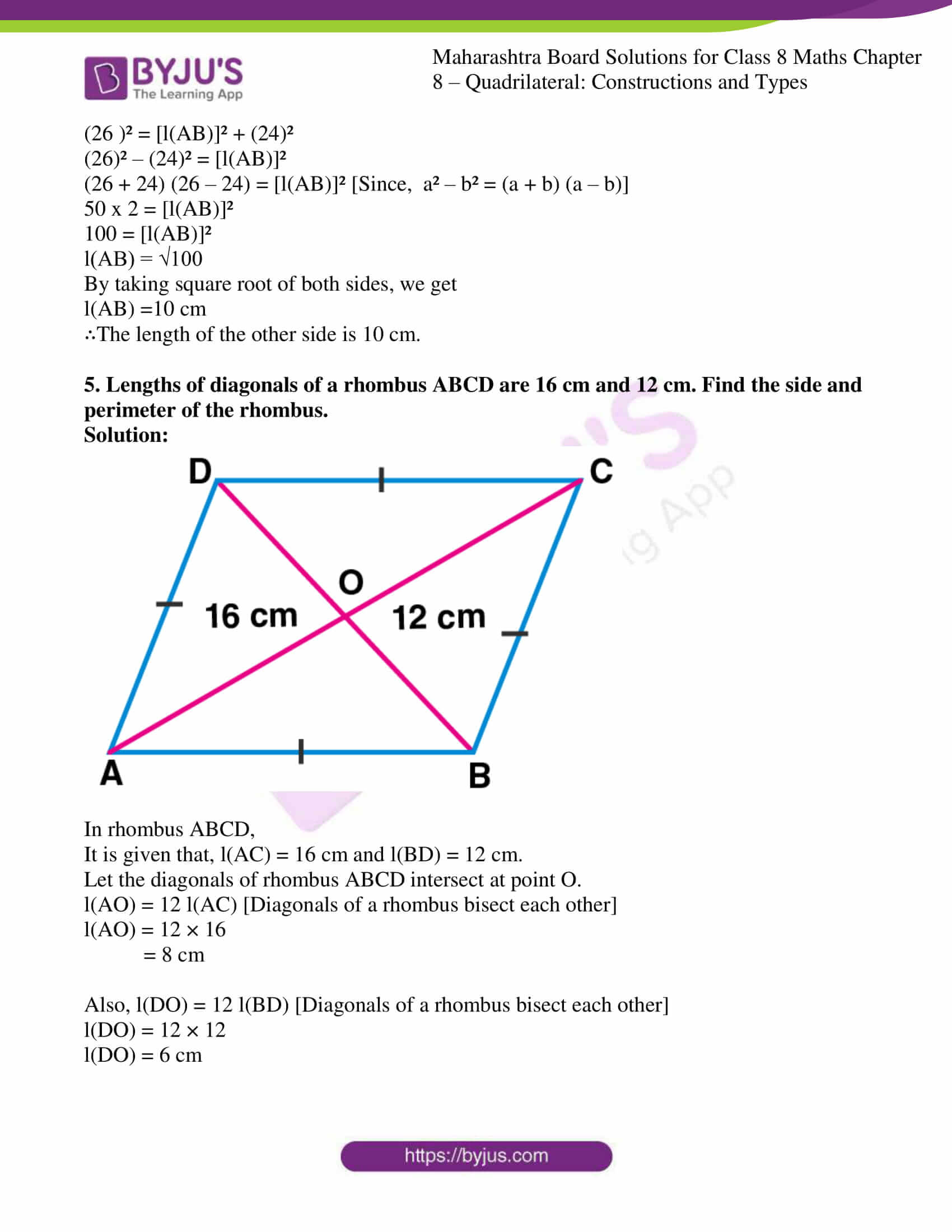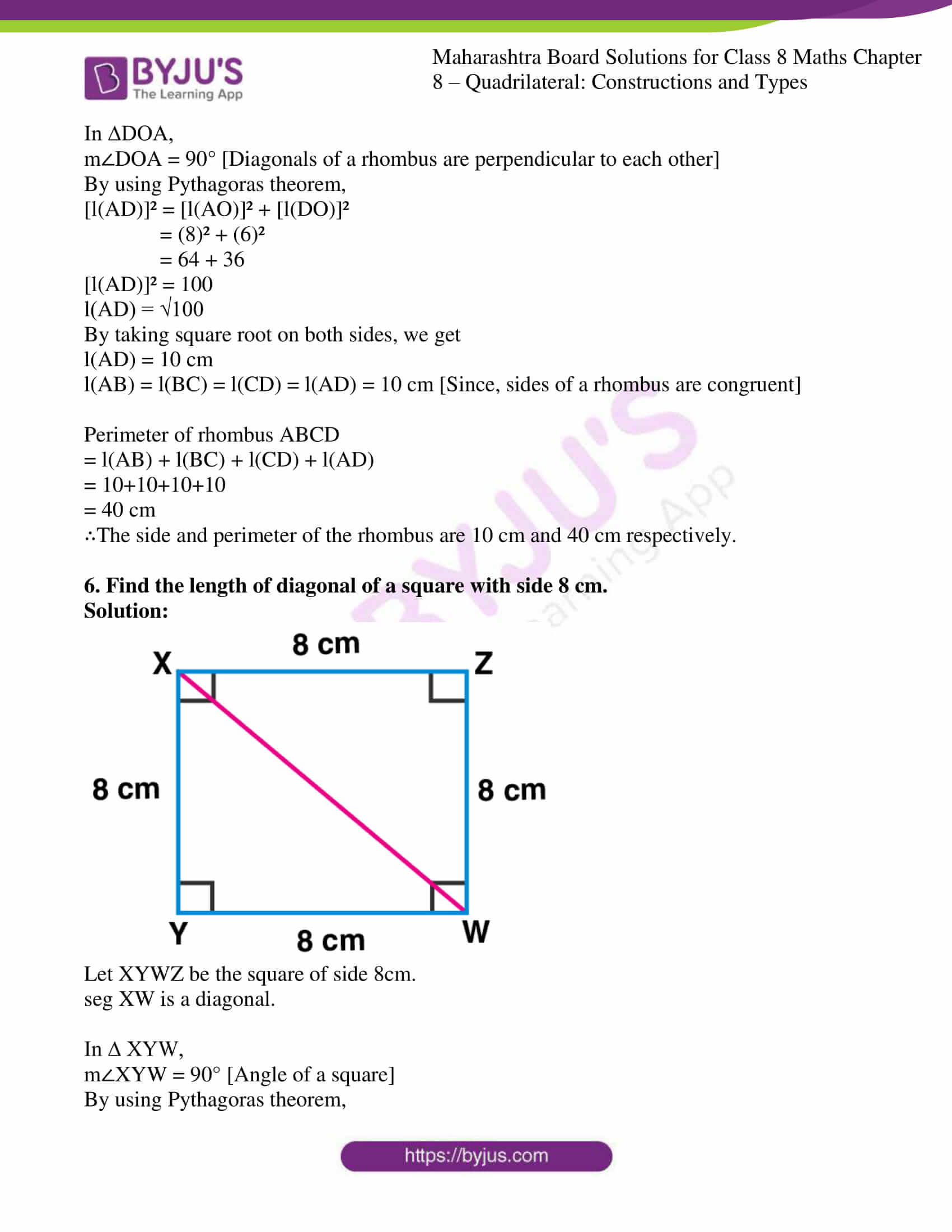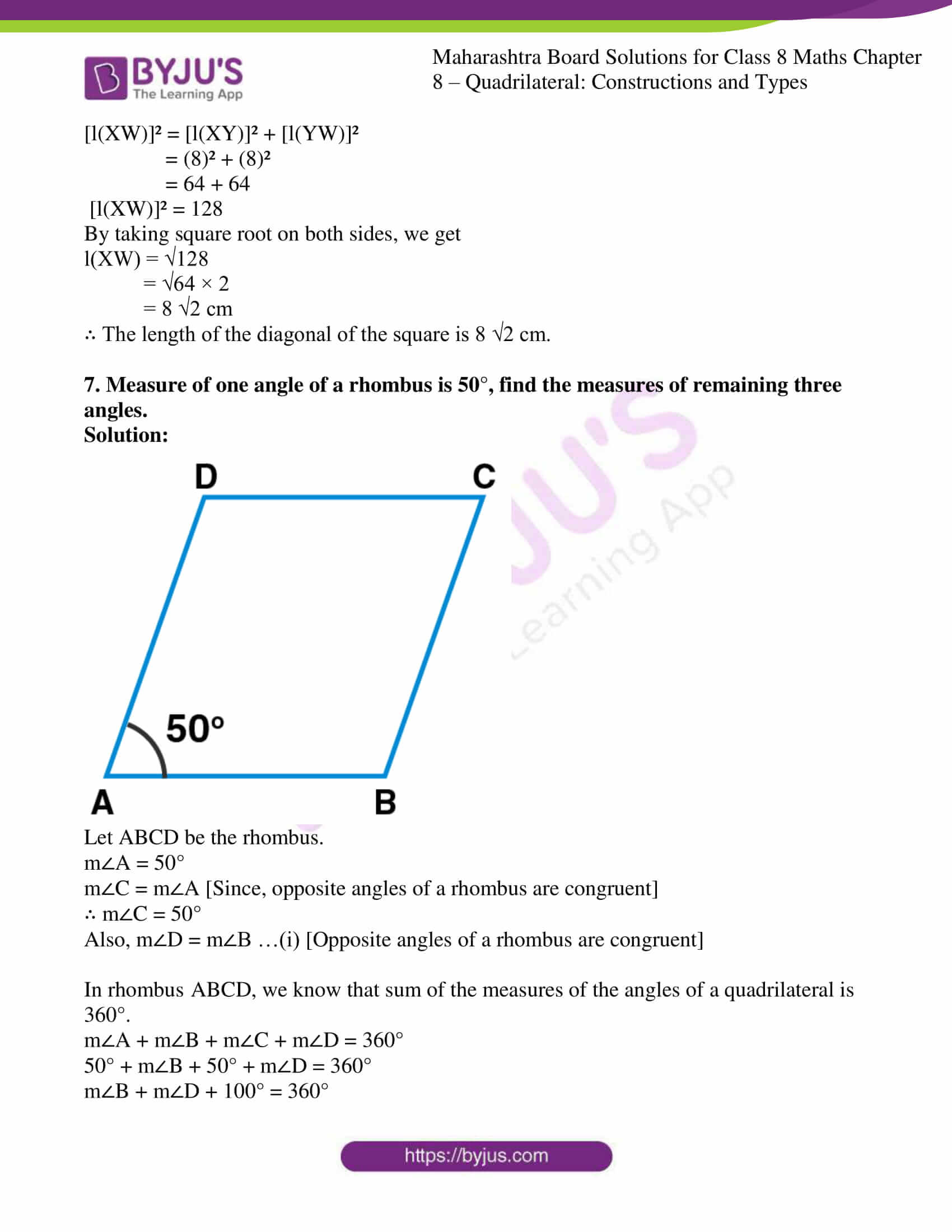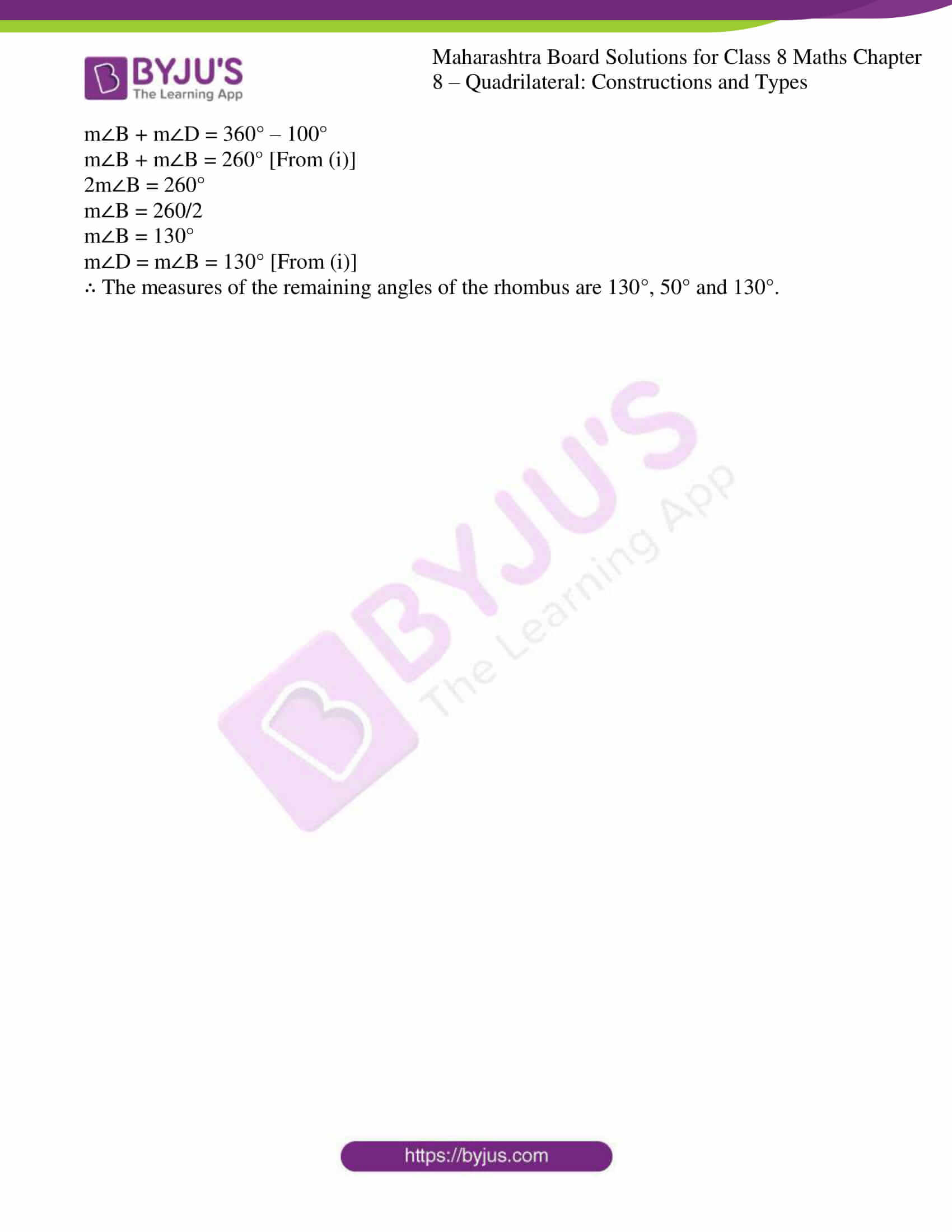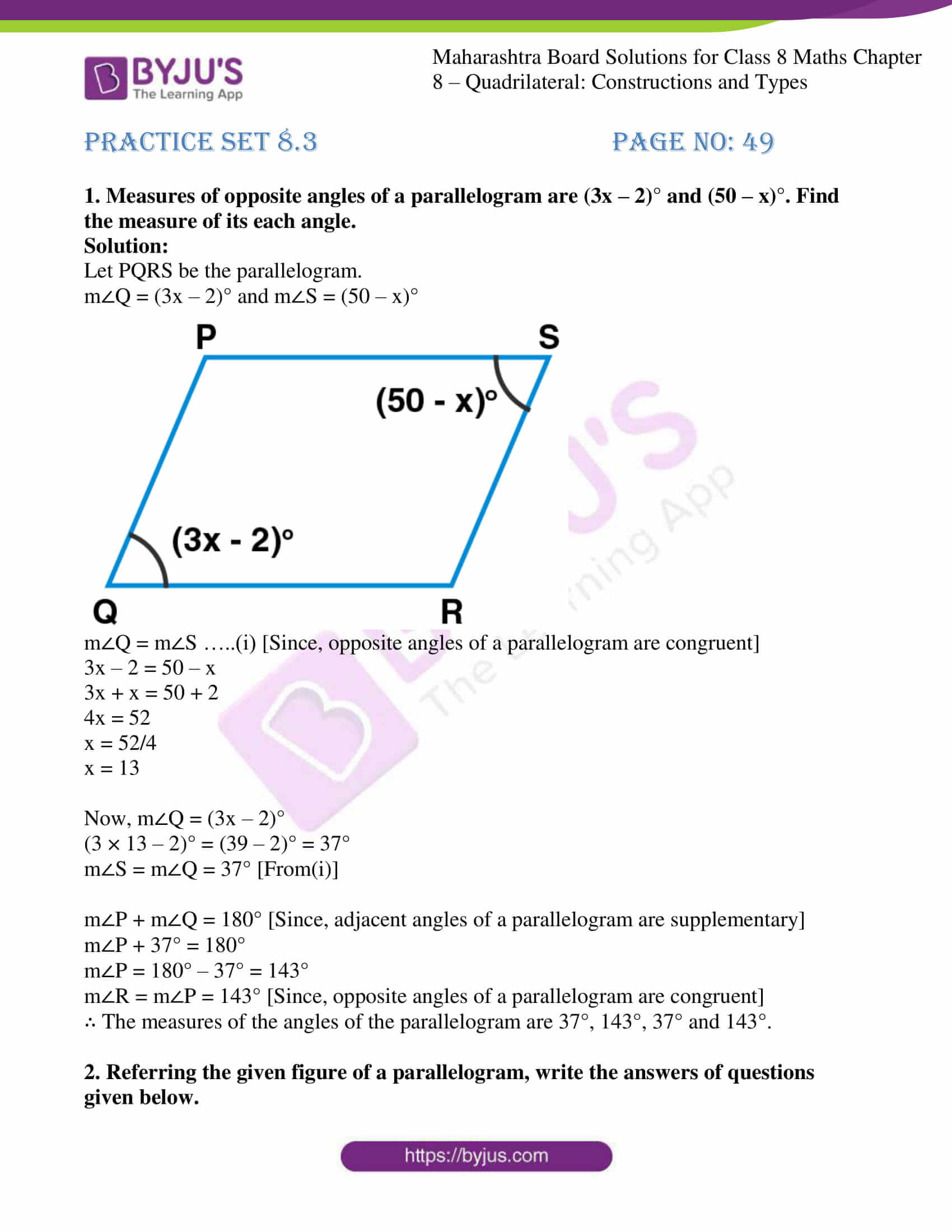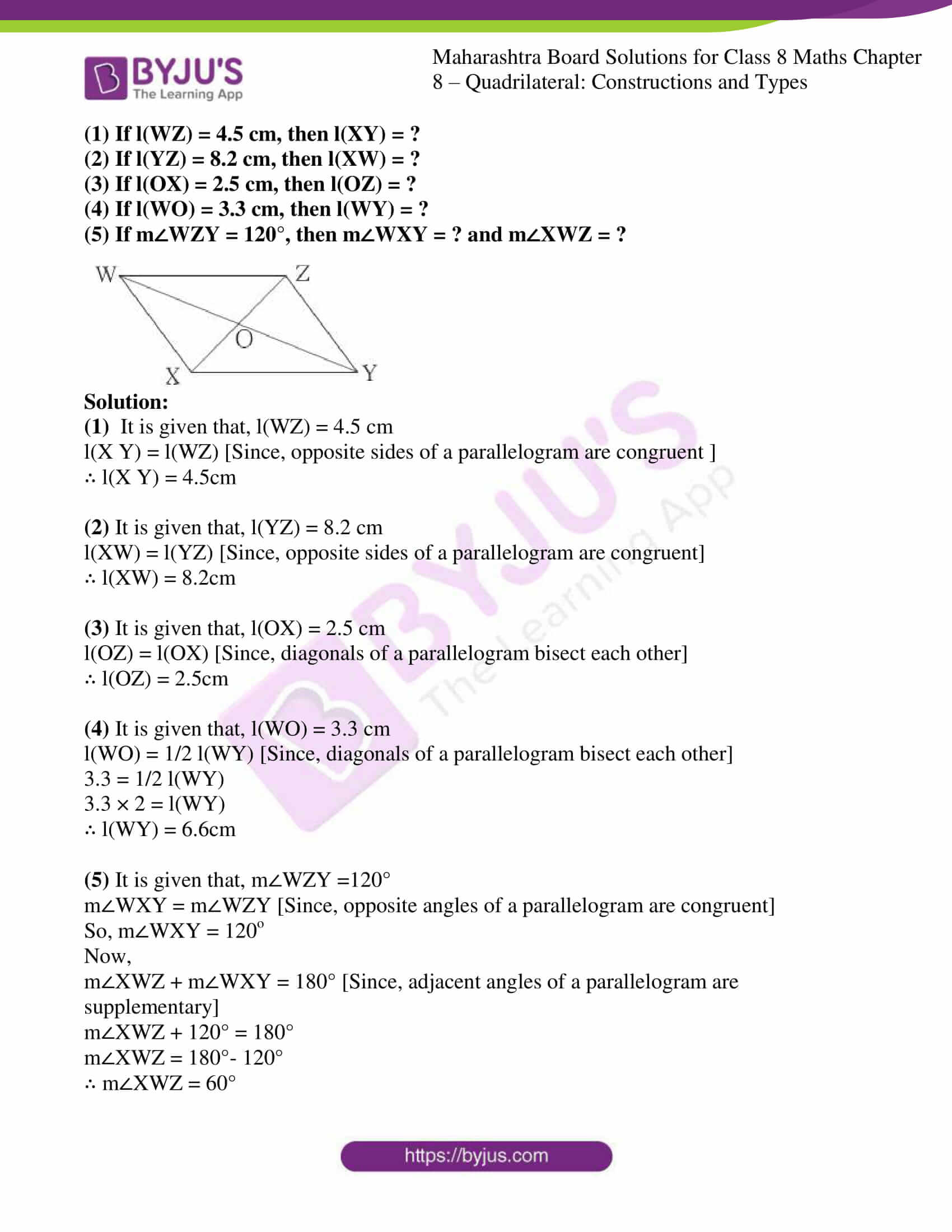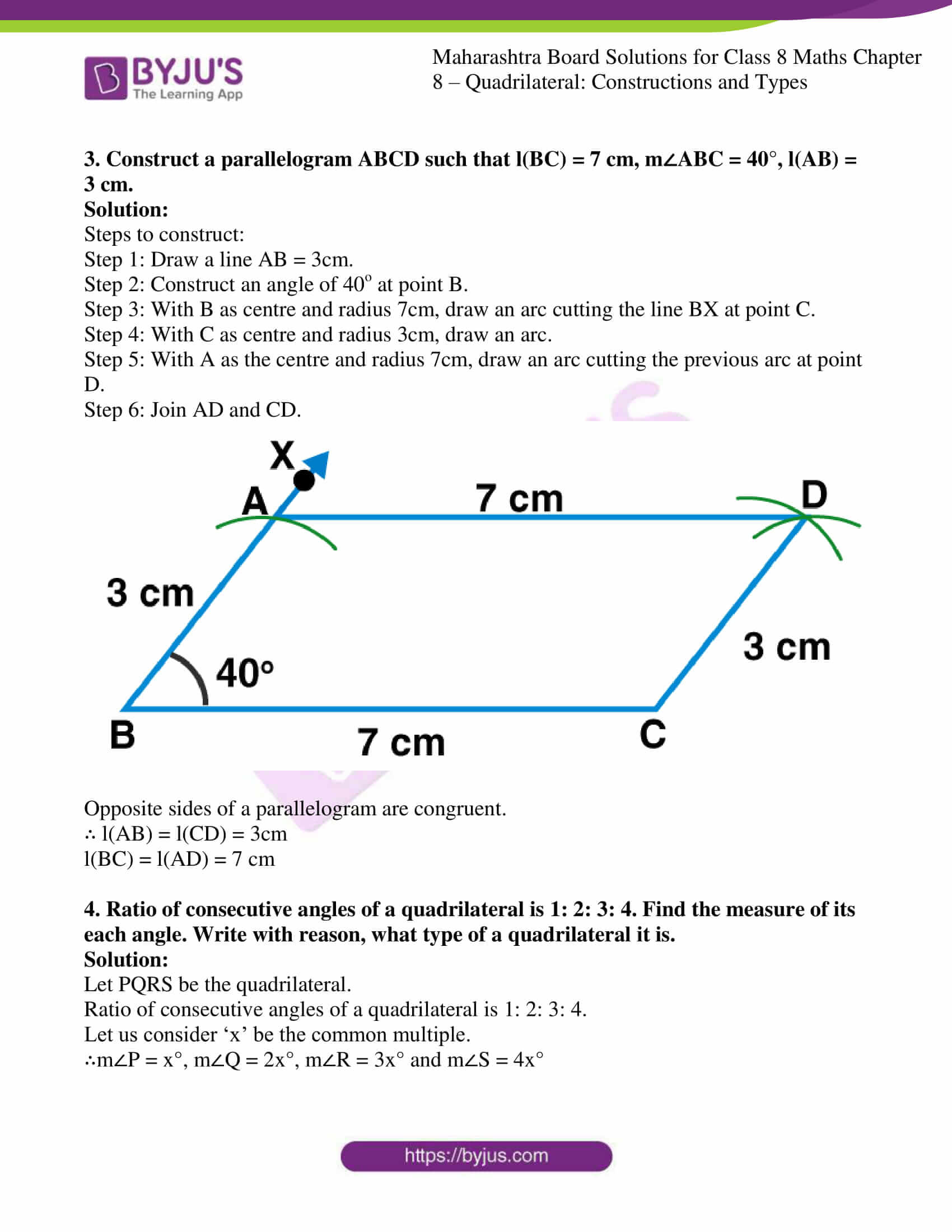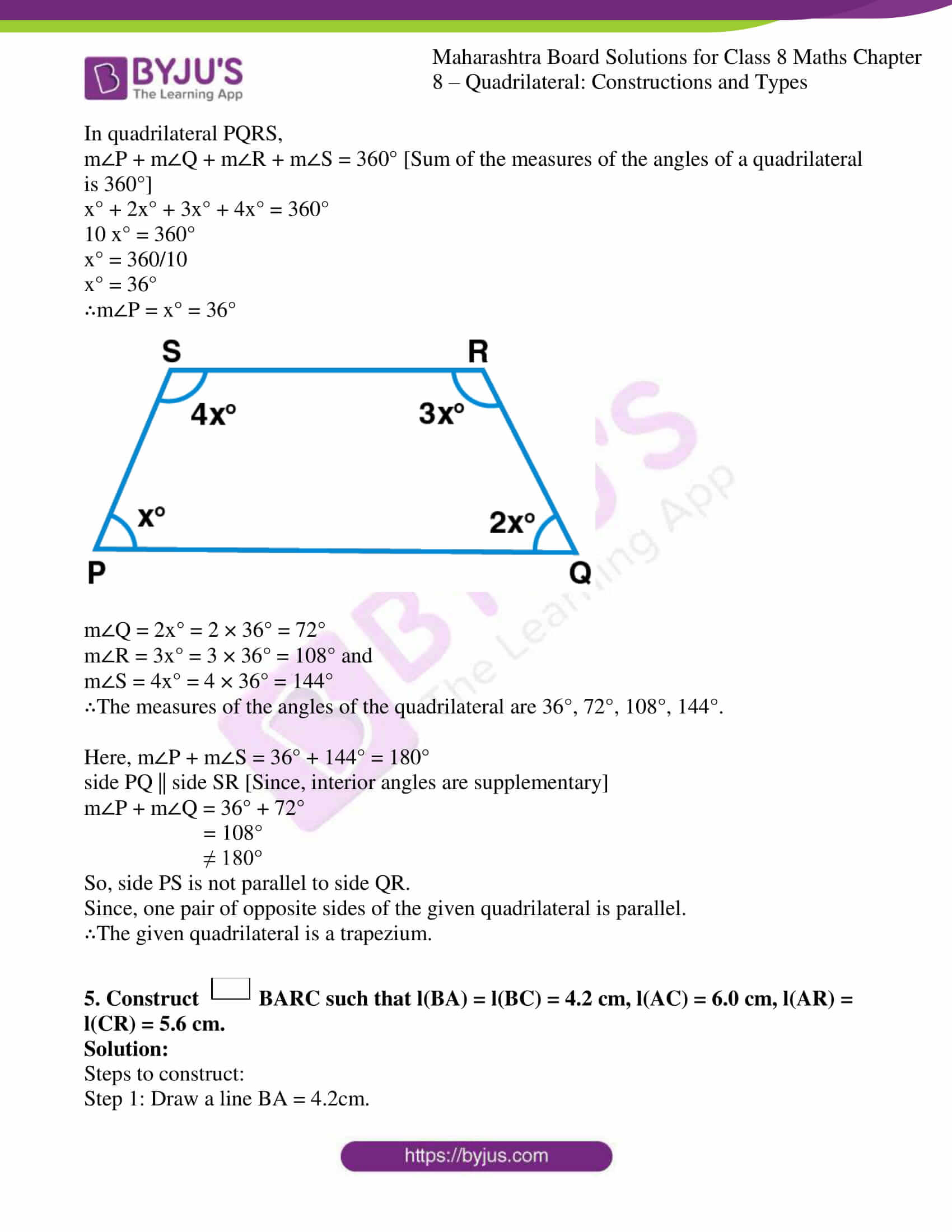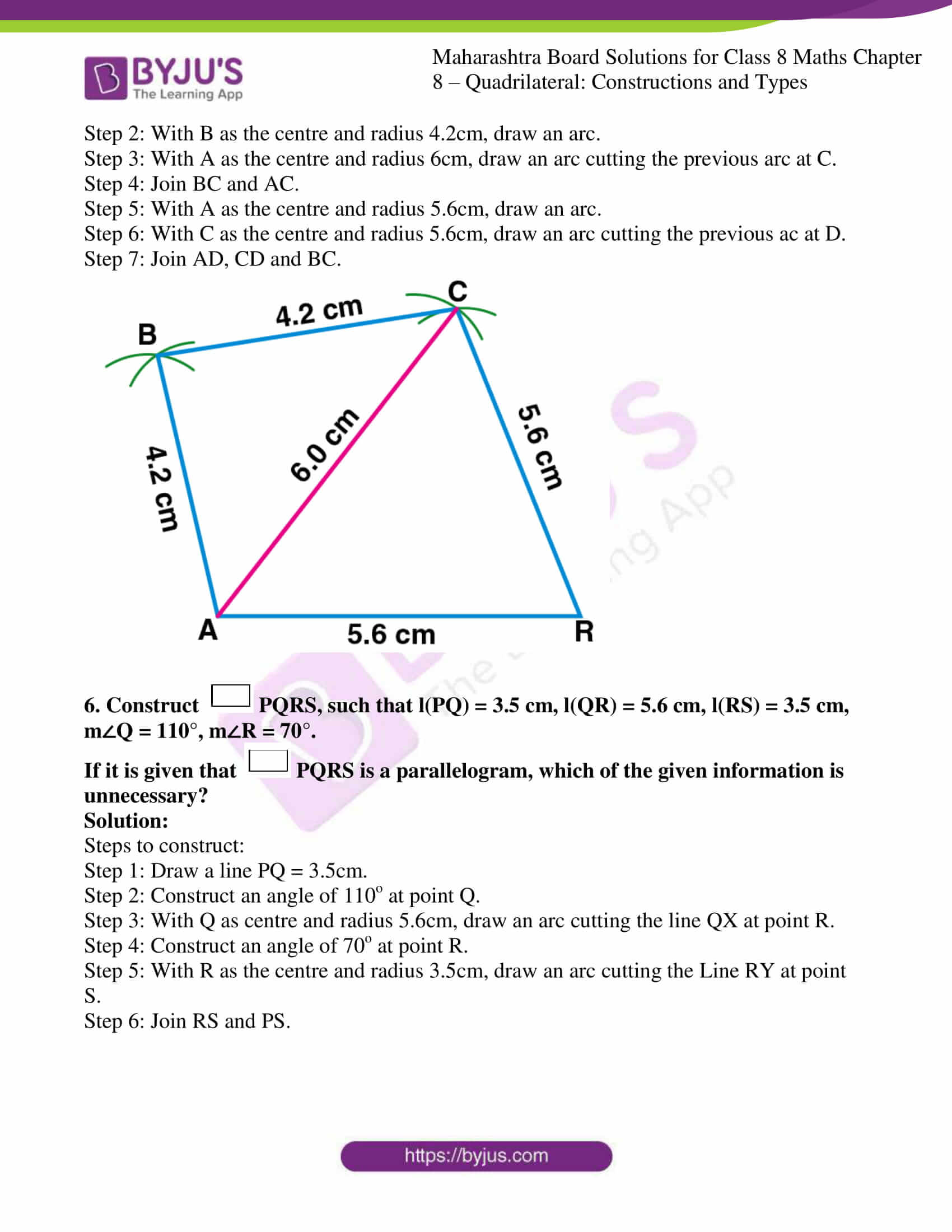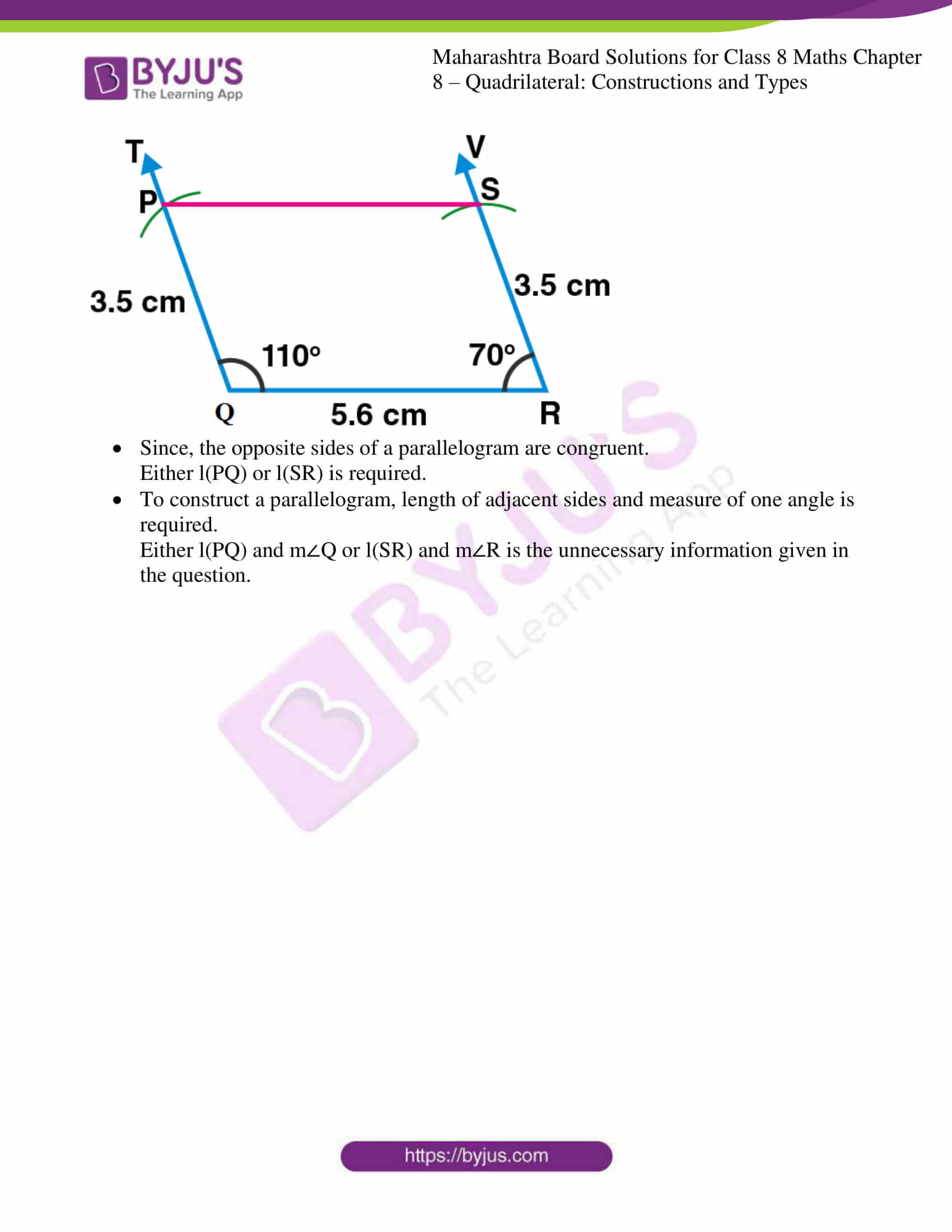### Access answers to Maharashtra Board Solutions For Class 8 Maths Part 1 Chapter 8- Quadrilateral: Constructions and Types.

Practice set 8.1 PAGE NO: 43

1. Construct the following quadrilaterals of given measures.

(1) In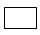MORE, l(MO) = 5.8 cm, l(OR) = 4.4 cm, m∠M = 58°, m∠O = 105°, m∠R = 90°.

(2) ConstructDEFG such that l(DE) = 4.5 cm, l(EF) = 6.5 cm, l(DG) = 5.5 cm, l(DF) = 7.2 cm, l(EG) = 7.8 cm.

(3) InABCD, l(AB) = 6.4 cm, l(BC) = 4.8 cm, m∠A = 70°, m∠B = 50°, m∠C = 140°.

(4) ConstructLMNO such that l(LM) = l(LO) = 6 cm, l(ON) = l(NM) = 4.5 cm, l(OM) = 7.5 cm.

Solution:

(1) InMORE, l(MO) = 5.8 cm, l(OR) = 4.4 cm, m∠M = 58°, m∠O = 105°, m∠R = 90°.

Step 1: Draw a line MO = 5.8cm.

Step 2: At point O, construct an angle of 105o. Such that it forms a line OX.

Step 3: At point M, construct an angle of 58o. Such that it forms a line MZ.

Step 4: With O as the centre and of radius 4.4cm, cut an arc on the line OX and mark that point as R.

Step 5: Now, at point R construct an angle of 90o. Such that it forms a line RY and the point E is formed intersecting on the line MZ.

Here, is the required MORE quadrilateral.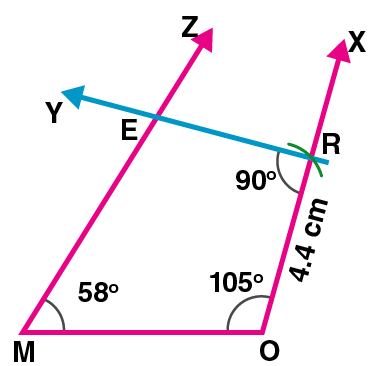(2) ConstructDEFG such that l(DE) = 4.5 cm, l(EF) = 6.5 cm, l(DG) = 5.5 cm, l(DF) = 7.2 cm, l(EG) = 7.8 cm.

Step 1: Draw a line DE = 4.5cm.

Step 2: With D as the centre and radius 7.2cm, draw an arc.

Step 3: With E as the centre and radius 6.5cm, draw an arc cutting the previous arc at F.

Step 4: Join EF and DF.

Step 5: With D as the centre and radius 5.5cm, draw an arc.

Step 6: With E as the centre and radius 7.8cm, draw an arc cutting the previous ac at G.

Step 7: Join DG, EG and GF.

Here, is the required DEFG quadrilateral.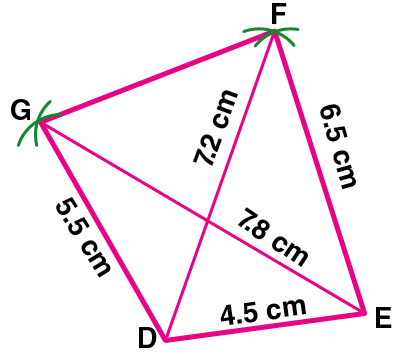(3) InABCD, l(AB) = 6.4 cm, l(BC) = 4.8 cm, m∠A = 70°, m∠B = 50°, m∠C = 140°.

Step 1: Draw a line AB = 6.4cm.

Step 2: construct an angle at point B of 50o

Step 3: With B as the centre and radius of 4.8cm, draw an arc cutting the line BX at point C.

Step 4: construct an angle of 140o at point C and name that line as Y.

Step 5: construct an angle of 70o at point A, such that line AZ and line CY intersect at point D.

Here, is the required ABCD quadrilateral.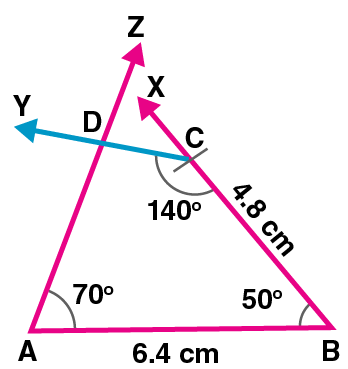(4) ConstructLMNO such that l(LM) = l(LO) = 6 cm, l(ON) = l(NM) = 4.5 cm, l(OM) = 7.5 cm.

Step 1: Draw a line LM = 6cm.

Step 2: With L as the centre and radius 6cm, draw an arc.

Step 3: With M as the centre and radius 7.5cm, draw an arc cutting the previous arc at O.

Step 4: Join OL and OL.

Step 5: With O as the centre and radius 4.5cm, draw an arc.

Step 6: With M as the centre and radius 4.5cm, draw an arc cutting the previous ac at N.

Step 7: Join ON, MN and OL.

Here, is the required LMNO quadrilateral.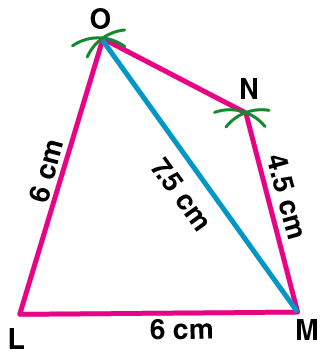Practice set 8.2 PAGE NO: 46

1. Draw a rectangle ABCD such that l(AB) = 6.0 cm and l(BC) = 4.5 cm.

Solution:

Steps to construct a rectangle:

Step 1: Draw a line AB = 6cm.

Step 2: Construct an angle of 90o at point B.

Step 3: With B as centre and radius 4.5cm, draw an arc cutting the line BX at point C.

Step 4: With C as centre and radius 6cm, draw an arc.

Step 5: With A as the centre and radius 4.5cm, draw an arc cutting the previous arc at point D.

Step 6: Join AD and CD.

Here, is the required ABCD rectangle.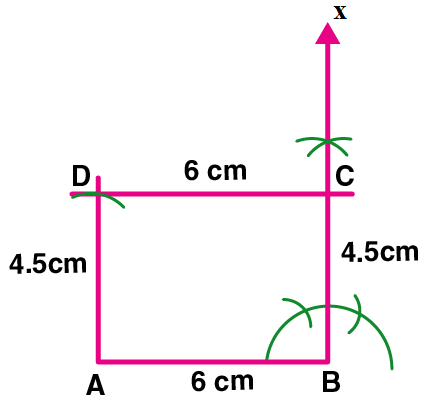2. Draw a square WXYZ with side 5.2 cm.

Solution:

Steps to construct a square:

Step 1: Draw a line WX = 5.2cm.

Step 2: Construct an angle of 90o at point X.

Step 3: With X as centre and radius 5.2cm, draw an arc cutting the line XP at point Y.

Step 4: With Y as centre and radius 5.2cm, draw an arc.

Step 5: With W as the centre and radius 5.2cm, draw an arc cutting the previous arc at point Z.

Step 6: Join YZ and WZ.

Here, is the required WXYZ square.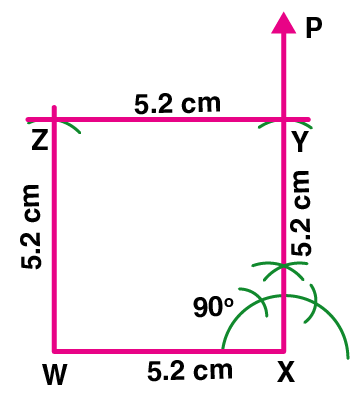3. Draw a rhombus KLMN such that its side is 4 cm and m∠K = 75°.

Solution:

Steps to construct a rhombus:

Step 1: Draw a line KL = 4cm.

Step 2: Construct an angle of 75o at point K.

Step 3: With K as centre and radius 4cm, draw an arc cutting the line KX at point N.

Step 4: With N as centre and radius 4cm, draw an arc.

Step 5: With L as the centre and radius 4cm, draw an arc cutting the previous arc at point M.

Step 6: Join LM and NM.

Here, is the required KLMN rhombus.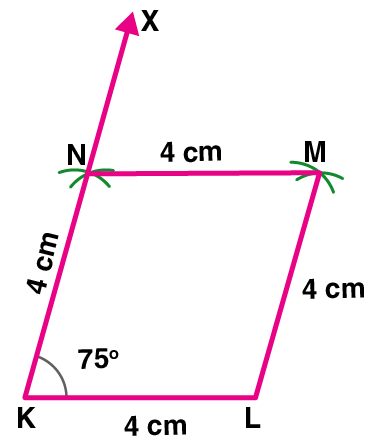4. If diagonal of a rectangle is 26 cm and one side is 24 cm, find the other side.

Solution: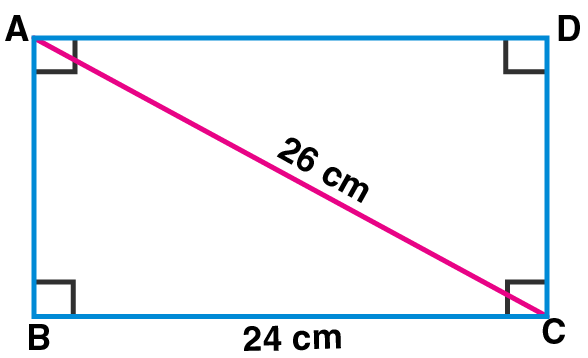Let ABCD be the rectangle.

l(BC) = 24cm, l(AC) = 26cm

In ∆ABC,

m∠ABC = 90° [Angle of a rectangle]

By using Pythagoras theorem,

[l(AC)]² = [l(AB)]2 + [l(BC)]²

(26 )² = [l(AB)]² + (24)²

(26)² – (24)² = [l(AB)]²

(26 + 24) (26 – 24) = [l(AB)]² [Since, a² – b² = (a + b) (a – b)]

50 x 2 = [l(AB)]²

100 = [l(AB)]²

l(AB) = √100

By taking square root of both sides, we get

l(AB) =10 cm

∴The length of the other side is 10 cm.

5. Lengths of diagonals of a rhombus ABCD are 16 cm and 12 cm. Find the side and perimeter of the rhombus.

Solution: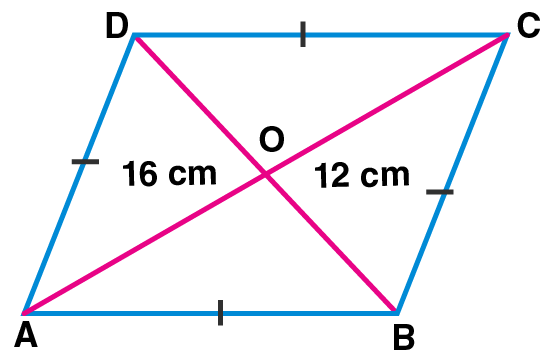In rhombus ABCD,

It is given that, l(AC) = 16 cm and l(BD) = 12 cm.

Let the diagonals of rhombus ABCD intersect at point O.

l(AO) = 12 l(AC) [Diagonals of a rhombus bisect each other]

l(AO) = 12 × 16

= 8 cm

Also, l(DO) = 12 l(BD) [Diagonals of a rhombus bisect each other]

l(DO) = 12 × 12

l(DO) = 6 cm

In ∆DOA,

m∠DOA = 90° [Diagonals of a rhombus are perpendicular to each other]

By using Pythagoras theorem,

= (8)² + (6)²

= 64 + 36

By taking square root on both sides, we get

l(AB) = l(BC) = l(CD) = l(AD) = 10 cm [Since, sides of a rhombus are congruent]

Perimeter of rhombus ABCD

= l(AB) + l(BC) + l(CD) + l(AD)

= 10+10+10+10

= 40 cm

∴The side and perimeter of the rhombus are 10 cm and 40 cm respectively.

6. Find the length of diagonal of a square with side 8 cm.

Solution: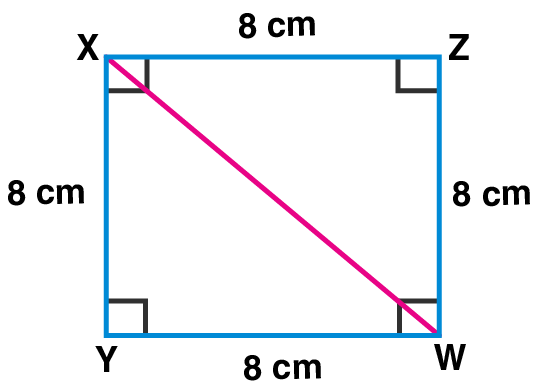Let XYWZ be the square of side 8cm.

seg XW is a diagonal.

In ∆ XYW,

m∠XYW = 90° [Angle of a square]

By using Pythagoras theorem,

[l(XW)]² = [l(XY)]² + [l(YW)]²

= (8)² + (8)²

= 64 + 64

[l(XW)]² = 128

By taking square root on both sides, we get

l(XW) = √128

= √64 × 2

= 8 √2 cm

∴ The length of the diagonal of the square is 8 √2 cm.

7. Measure of one angle of a rhombus is 50°, find the measures of remaining three angles.

Solution: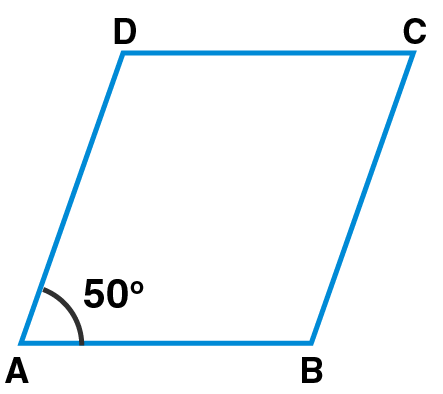Let ABCD be the rhombus.

m∠A = 50°

m∠C = m∠A [Since, opposite angles of a rhombus are congruent]

∴ m∠C = 50°

Also, m∠D = m∠B …(i) [Opposite angles of a rhombus are congruent]

In rhombus ABCD, we know that sum of the measures of the angles of a quadrilateral is 360°.

m∠A + m∠B + m∠C + m∠D = 360°

50° + m∠B + 50° + m∠D = 360°

m∠B + m∠D + 100° = 360°

m∠B + m∠D = 360° – 100°

m∠B + m∠B = 260° [From (i)]

2m∠B = 260°

m∠B = 260/2

m∠B = 130°

m∠D = m∠B = 130° [From (i)]

∴ The measures of the remaining angles of the rhombus are 130°, 50° and 130°.

Practice set 8.3 PAGE NO: 49

1. Measures of opposite angles of a parallelogram are (3x – 2)° and (50 – x)°. Find the measure of its each angle.

Solution:

Let PQRS be the parallelogram.

m∠Q = (3x – 2)° and m∠S = (50 – x)°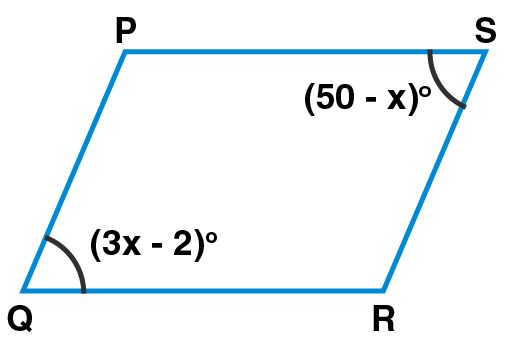m∠Q = m∠S …..(i) [Since, opposite angles of a parallelogram are congruent]

3x – 2 = 50 – x

3x + x = 50 + 2

4x = 52

x = 52/4

x = 13

Now, m∠Q = (3x – 2)°

(3 × 13 – 2)° = (39 – 2)° = 37°

m∠S = m∠Q = 37° [From(i)]

m∠P + m∠Q = 180° [Since, adjacent angles of a parallelogram are supplementary]

m∠P + 37° = 180°

m∠P = 180° – 37° = 143°

m∠R = m∠P = 143° [Since, opposite angles of a parallelogram are congruent]

∴ The measures of the angles of the parallelogram are 37°, 143°, 37° and 143°.

2. Referring the given figure of a parallelogram, write the answers of questions given below.
(1) If l(WZ) = 4.5 cm, then l(XY) = ?
(2) If l(YZ) = 8.2 cm, then l(XW) = ?
(3) If l(OX) = 2.5 cm, then l(OZ) = ?
(4) If l(WO) = 3.3 cm, then l(WY) = ?
(5) If m∠WZY = 120°, then m∠WXY = ? and m∠XWZ = ?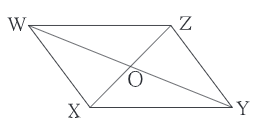Solution:

(1) It is given that, l(WZ) = 4.5 cm

l(X Y) = l(WZ) [Since, opposite sides of a parallelogram are congruent ]

∴ l(X Y) = 4.5cm

(2) It is given that, l(YZ) = 8.2 cm

l(XW) = l(YZ) [Since, opposite sides of a parallelogram are congruent]

∴ l(XW) = 8.2cm

(3) It is given that, l(OX) = 2.5 cm

l(OZ) = l(OX) [Since, diagonals of a parallelogram bisect each other]

∴ l(OZ) = 2.5cm

(4) It is given that, l(WO) = 3.3 cm

l(WO) = 1/2 l(WY) [Since, diagonals of a parallelogram bisect each other]

3.3 = 1/2 l(WY)

3.3 × 2 = l(WY)

∴ l(WY) = 6.6cm

(5) It is given that, m∠WZY =120°

m∠WXY = m∠WZY [Since, opposite angles of a parallelogram are congruent]

So, m∠WXY = 120o

Now,

m∠XWZ + m∠WXY = 180° [Since, adjacent angles of a parallelogram are supplementary]

m∠XWZ + 120° = 180°

m∠XWZ = 180°- 120°

∴ m∠XWZ = 60°

3. Construct a parallelogram ABCD such that l(BC) = 7 cm, m∠ABC = 40°, l(AB) = 3 cm.

Solution:

Steps to construct:

Step 1: Draw a line AB = 3cm.

Step 2: Construct an angle of 40o at point B.

Step 3: With B as centre and radius 7cm, draw an arc cutting the line BX at point C.

Step 4: With C as centre and radius 3cm, draw an arc.

Step 5: With A as the centre and radius 7cm, draw an arc cutting the previous arc at point D.

Step 6: Join AD and CD.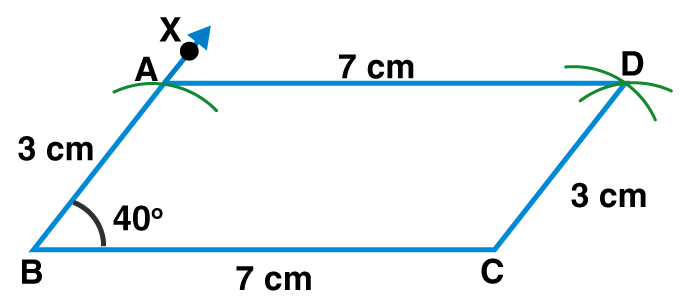Opposite sides of a parallelogram are congruent.

∴ l(AB) = l(CD) = 3cm

l(BC) = l(AD) = 7 cm

4. Ratio of consecutive angles of a quadrilateral is 1: 2: 3: 4. Find the measure of its each angle. Write with reason, what type of a quadrilateral it is.

Solution:

Ratio of consecutive angles of a quadrilateral is 1: 2: 3: 4.

Let us consider ‘x’ be the common multiple.

∴m∠P = x°, m∠Q = 2x°, m∠R = 3x° and m∠S = 4x°

m∠P + m∠Q + m∠R + m∠S = 360° [Sum of the measures of the angles of a quadrilateral is 360°]

x° + 2x° + 3x° + 4x° = 360°

10 x° = 360°

x° = 360/10

x° = 36°

∴m∠P = x° = 36°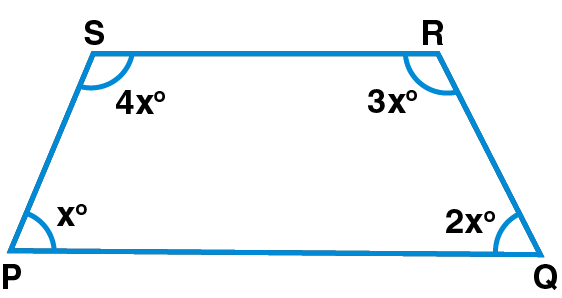m∠Q = 2x° = 2 × 36° = 72°

m∠R = 3x° = 3 × 36° = 108° and

m∠S = 4x° = 4 × 36° = 144°

∴The measures of the angles of the quadrilateral are 36°, 72°, 108°, 144°.

Here, m∠P + m∠S = 36° + 144° = 180°

side PQ || side SR [Since, interior angles are supplementary]

m∠P + m∠Q = 36° + 72°

= 108°

≠ 180°

So, side PS is not parallel to side QR.

Since, one pair of opposite sides of the given quadrilateral is parallel.

∴The given quadrilateral is a trapezium.

5. ConstructBARC such that l(BA) = l(BC) = 4.2 cm, l(AC) = 6.0 cm, l(AR) = l(CR) = 5.6 cm.

Solution:

Steps to construct:

Step 1: Draw a line BA = 4.2cm.

Step 2: With B as the centre and radius 4.2cm, draw an arc.

Step 3: With A as the centre and radius 6cm, draw an arc cutting the previous arc at C.

Step 4: Join BC and AC.

Step 5: With A as the centre and radius 5.6cm, draw an arc.

Step 6: With C as the centre and radius 5.6cm, draw an arc cutting the previous ac at D.

Step 7: Join AD, CD and BC.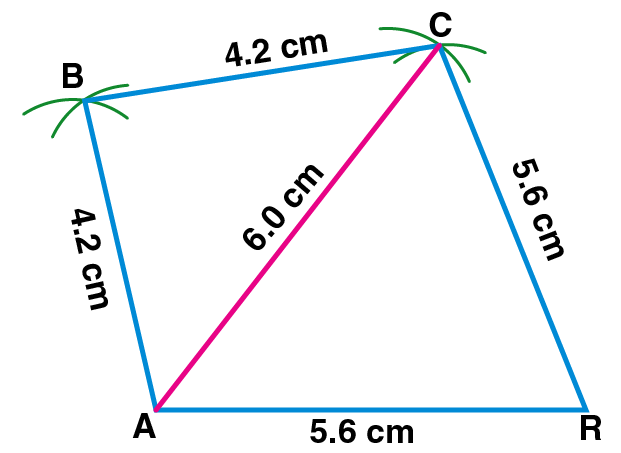6. ConstructPQRS, such that l(PQ) = 3.5 cm, l(QR) = 5.6 cm, l(RS) = 3.5 cm, m∠Q = 110°, m∠R = 70°.

If it is given thatPQRS is a parallelogram, which of the given information is unnecessary?

Solution:

Steps to construct:

Step 1: Draw a line PQ = 3.5cm.

Step 2: Construct an angle of 110o at point Q.

Step 3: With Q as centre and radius 5.6cm, draw an arc cutting the line QX at point R.

Step 4: Construct an angle of 70o at point R.

Step 5: With R as the centre and radius 3.5cm, draw an arc cutting the Line RY at point S.

Step 6: Join RS and PS.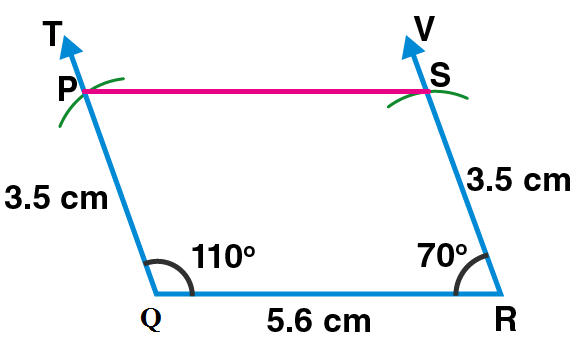• Since, the opposite sides of a parallelogram are congruent.Either l(PQ) or l(SR) is required.
• To construct a parallelogram, length of adjacent sides and measure of one angle is required.Either l(PQ) and m∠Q or l(SR) and m∠R is the unnecessary information given in the question.

In this chapter, we shall discuss concepts related to Construction of Quadrilaterals, with examples for better understanding. MSBSHSE Solutions contains all the solutions to the Maths problems provided in the textbook. Students can refer to these solutions and score good marks in the board exams.

## Frequently Asked Questions on Maharashtra State Board Solutions for Class 8 Maths Chapter 8 Quadrilaterals: Constructions and Types

### Why are these Maharashtra State Board Class 8 Maths Chapter 8 Quadrilaterals: Constructions and Types Solutions important?

Maharashtra State Board Class 8 Maths Chapter 8 Quadrilaterals: Constructions and Types Solutions helps every student to easily comprehend the concept into their minds and clarify their doubts in a few seconds. The solutions are very self-explanatory and encourges the students to innovate and explore in Maths. Also, board exam aspirants can assess their learning abilities with these solutions.

### How do we access these solutions?

Maharashtra State Board Solutions for Class 8 Maths Chapter 8 Quadrilaterals: Constructions and Types are available online as scrollable PDF online and the solutions and questions are also given directly on the webpage.  We have also provided the PDF clickable links for those who wish to download the PDF format for future reference.

### How to make the most of Maharashtra State Board Class 8 Maths Chapter 8 Solutions?

Students are advised to refer to the questions from the Maharashtra State Board Class 8 Maths Chapter 8 Solutions and solve them first before going back to check the answers. This will help the students to self analyse their preparation level and also to identify the mistakes, rectify them and avoid them for the exams. Timing the process also helps to manage time in a better way.